# Lesson plan

Installation of the new LedBorg software
Lesson 1. Our first Python example
Lesson 2. Simplifying the code
Lesson 3. Produce a sequence of colours
Lesson 4. Taking user input
Lesson 5. Brightness levels (PWM)
Lesson 6. A simple dialog (GUI)
Lesson 7. Using a pre-made dialog

## Taking user input

With this next script we will take user input from the terminal, then use the input to set the colour of LedBorg.
Before we can write this script, we need to learn a couple of new techniques: if blocks and while loops.

### The if block

A common problem which comes up when writing scripts is the need to do something depending on some condition.
In most programming languages we have a tool known as an `if` block which allows us to do this.

For example, if I want to show the user an error if a temperature variable is out of range, how can I do it?
We can test this type of condition by using an if block like this:
```if temp > 50.0:
print 'Too high!'```
When the condition `temp > 50.0` is true, the lines indented below it are run.
If the condition is false the lines indented below are not run.

The if block can be extended to test more conditions with `elif` (shorthand for else if) and `else` as shown below:
```if temp > 50.0:
print 'Too high!'
elif temp < 20.0:
print 'Too low!'
else:
print 'Just right.'```
The if block tests the `temp > 50` condition first, if it is true, then ‘too high’ is printed.
If the first condition is false, the if block moves on to the next condition, `temp < 20`, if it is true then ‘too low’ is printed instead.
If both conditions are false the if block uses the unconditional else lines, which will mean ‘just right’ is printed. The elif and else sections are optional, you may also use as many elif sections as you like.

### The while loop

The for loop we encountered in the previous lesson is suitable if we have a list of items to go through, but what if we want to keep going until a condition tests false?
For this we need a while loop.
```a = 0
while a < 3:
print a
a += 1```
This will print the following:
```0
1
2```
When we reach the while loop, the lines indented below the while statement will be run as long as the condition, `a < 3` remains true.
The `a += 1` is shorthand for `a = a + 1`, the same shorthand works with the standard mathematical operators: + - * /
Each time we run through the lines in the loop, the variable a is incremented by the `a += 1` line, in this example it causes the condition to become false and stop the loop running.

We can also make a loop run forever by giving a condition which never tests false:
```while True:
print 'Badger'
time.sleep(0.5)```
This will print the word Badger to the terminal twice a second.

### Setting up our loop

We can modify the lesson3.py script to make our new script, start by copying the old script:
`cp lesson3.py lesson4.py`
Then open up the new script in your editor and remove lines 26 onwards (the script should now finish after the LedBorgOff function definition).

Before we can check what colour we want LedBorg to show, we need to ask the user what colour they want:
```# Get a colour name from the user, loop while they enter names
colourName = raw_input('Next colour? ')
while len(colourName) > 0:
# Convert the user entry to upper case
colourName = colourName.upper()
```
The `raw_input` function gets text from the keyboard until the user presses ENTER.
The condition we have used for the while loop will cause it to end when the user gives us a blank line.
It does this by using the `len` function to work out how many characters are in the line of text.

The call to the `.upper` function takes the colourName variable and makes all of the characters upper-cased.
We do this to make the if block conditions simpler, the following inputs:
`red` / `RED` / `Red` / `rEd` / `ReD`
all become `RED`, which is easier to check for than several possibilities.

### The selection of colours:

Here are the colour names we will use:
Red Green Blue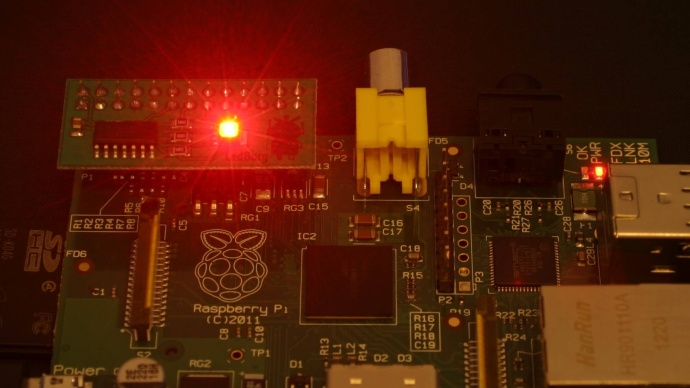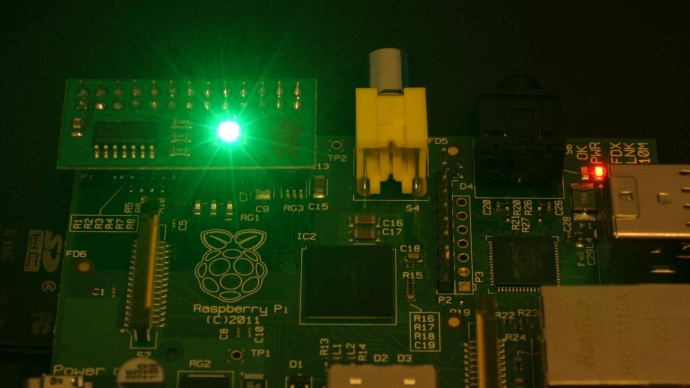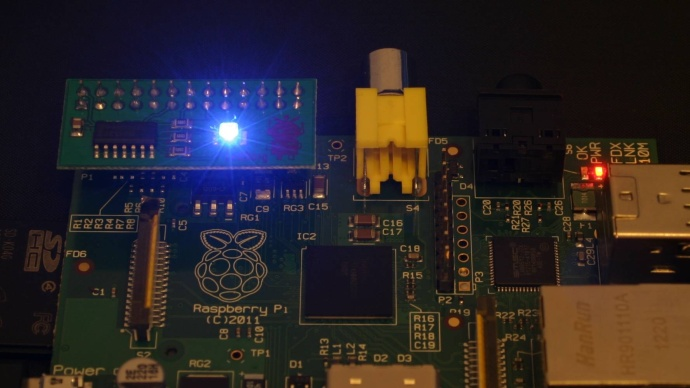Yellow Cyan Magenta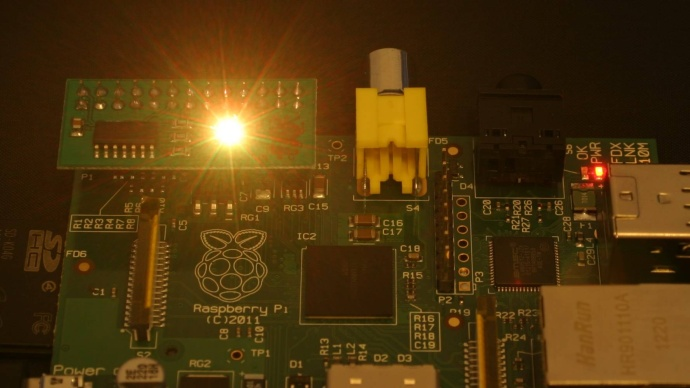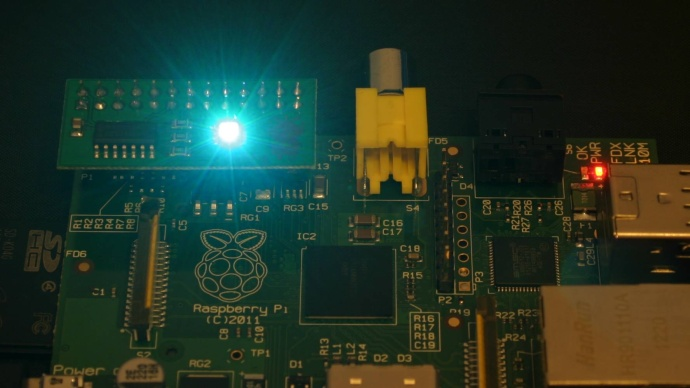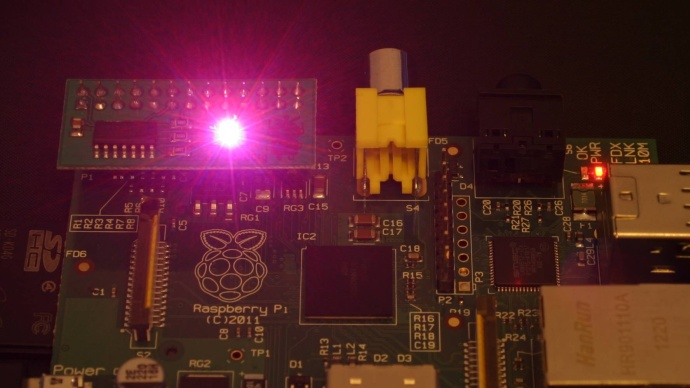White Black Off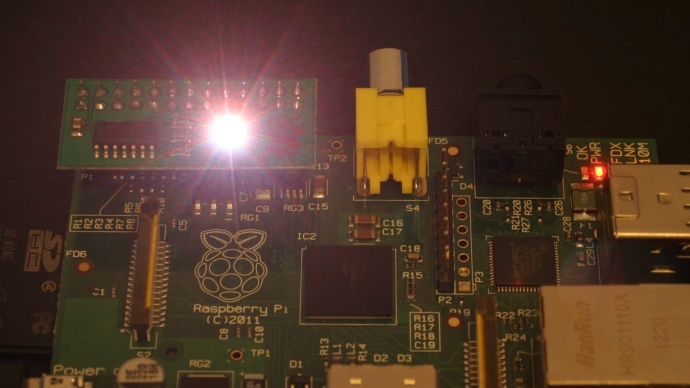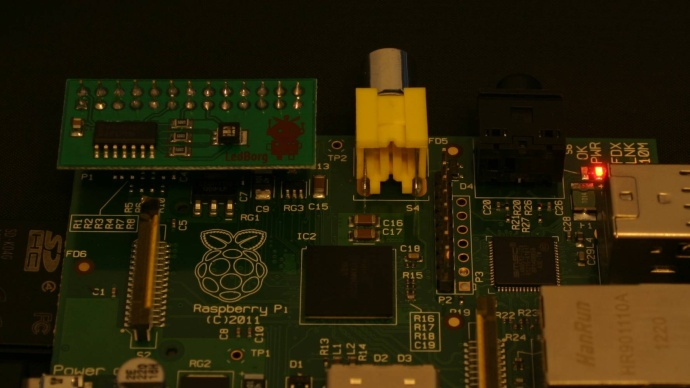As you can see above one nice thing about this if block is that it allows us to produce the same result (`0, 0, 0`) from more than one input (black or off).
The completed if-block looks like this:
```    # Work out which colour we want
if colourName == 'RED':
SetLedBorg(1, 0, 0)
elif colourName == 'GREEN':
SetLedBorg(0, 1, 0)
elif colourName == 'BLUE':
SetLedBorg(0, 0, 1)
elif colourName == 'YELLOW':
SetLedBorg(1, 1, 0)
elif colourName == 'CYAN':
SetLedBorg(0, 1, 1)
elif colourName == 'MAGENTA':
SetLedBorg(1, 0, 1)
elif colourName == 'WHITE':
SetLedBorg(1, 1, 1)
elif colourName == 'BLACK':
SetLedBorg(0, 0, 0)
elif colourName == 'OFF':
SetLedBorg(0, 0, 0)
else:
print 'I do not recognise that colour name'
```
Note the lines belonging to each condition are indented twice now, once to say they belong to the while loop and a second time to say they belong to the related condition.
Using this nesting allows us to make more complicated programs, for example:
• ifs inside ifs
• loops inside ifs
• ifs inside loops
• loops inside loops
• ifs inside functions
• loops inside functions

### Completing the loop

At the moment the script would set the option we choose when asked, then keep running setting the same colour.
In order for the script to be usable we need to ask the user for another colour, the same way we did before:
```    # Get the next colour name from the user
colourName = raw_input('Next colour? ')
```
Note that it is indented this time so that the line is run each time around the while loop.

### The complete script

The completed script can be downloaded directly to the Raspberry Pi using:
`wget -O lesson4.py http://www.piborg.org/downloads/ledborg-new/lesson4.txt`
Remember we make the script executable with:
`chmod +x lesson4.py`
and run it with:
`sudo ./lesson4.py`
```#!/usr/bin/env python

# Import the library functions we need
import time
import wiringpi2 as wiringpi
wiringpi.wiringPiSetup()

# Setup the LedBorg GPIO pins
PIN_RED = 0
PIN_GREEN = 2
PIN_BLUE = 3
wiringpi.pinMode(PIN_RED, wiringpi.GPIO.OUTPUT)
wiringpi.pinMode(PIN_GREEN, wiringpi.GPIO.OUTPUT)
wiringpi.pinMode(PIN_BLUE, wiringpi.GPIO.OUTPUT)

# A function to set the LedBorg colours
def SetLedBorg(red, green, blue):
wiringpi.digitalWrite(PIN_RED, red)
wiringpi.digitalWrite(PIN_GREEN, green)
wiringpi.digitalWrite(PIN_BLUE, blue)

# A function to turn the LedBorg off
def LedBorgOff():
SetLedBorg(0, 0, 0)

# Get a colour name from the user, loop while they enter names
colourName = raw_input('Next colour? ')
while len(colourName) > 0:
# Convert the user entry to upper case
colourName = colourName.upper()
# Work out which colour we want
if colourName == 'RED':
SetLedBorg(1, 0, 0)
elif colourName == 'GREEN':
SetLedBorg(0, 1, 0)
elif colourName == 'BLUE':
SetLedBorg(0, 0, 1)
elif colourName == 'YELLOW':
SetLedBorg(1, 1, 0)
elif colourName == 'CYAN':
SetLedBorg(0, 1, 1)
elif colourName == 'MAGENTA':
SetLedBorg(1, 0, 1)
elif colourName == 'WHITE':
SetLedBorg(1, 1, 1)
elif colourName == 'BLACK':
SetLedBorg(0, 0, 0)
elif colourName == 'OFF':
SetLedBorg(0, 0, 0)
else:
print 'I do not recognise that colour name'
# Get the next colour name from the user
colourName = raw_input('Next colour? ')
```

Continue to lesson 5. Brightness levels (PWM)